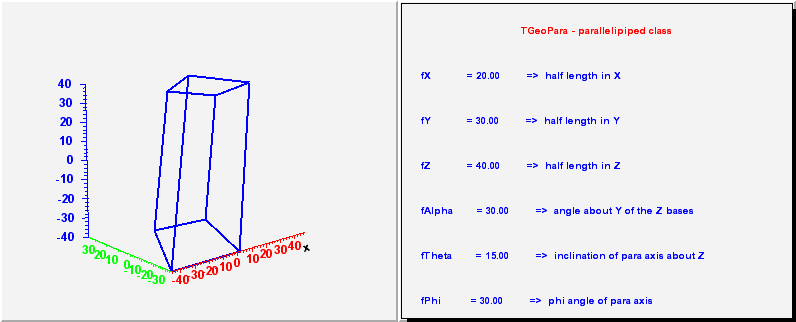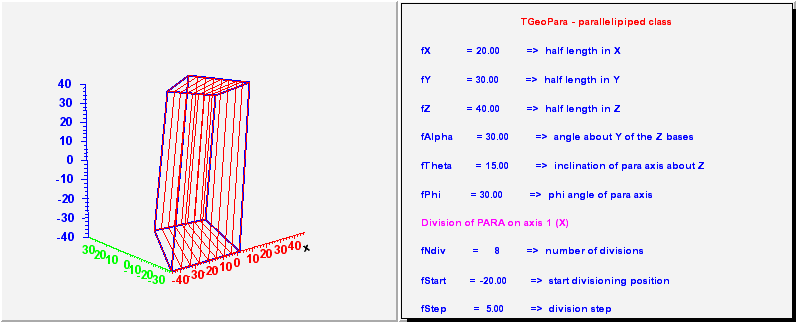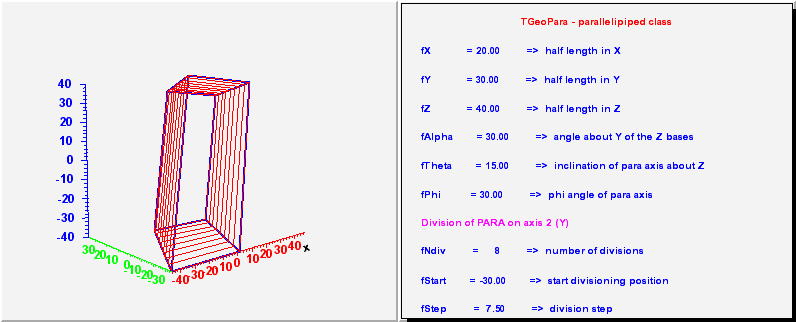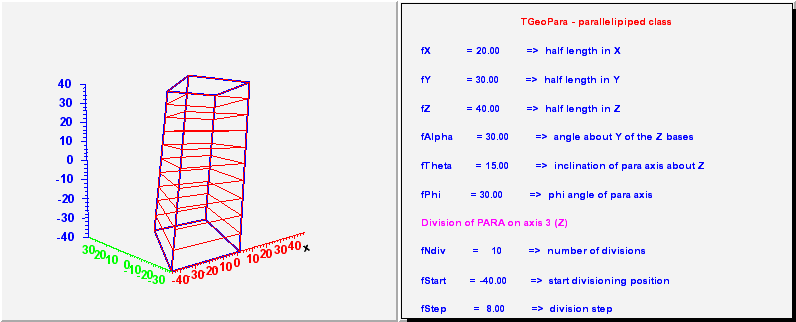library: libGeom#include "TGeoPara.h"

# TGeoPara

class description - source file - inheritance tree (.pdf)

## class TGeoPara : public TGeoBBox

Inheritance Chart:
 TObject
<-
TNamed
<-
TGeoShape
<-
TGeoBBox
<-
TGeoPara
```
public:
TGeoPara()
TGeoPara(Double_t dx, Double_t dy, Double_t dz, Double_t alpha, Double_t theta, Double_t phi)
TGeoPara(const char* name, Double_t dx, Double_t dy, Double_t dz, Double_t alpha, Double_t theta, Double_t phi)
TGeoPara(Double_t* param)
TGeoPara(const TGeoPara&)
virtual ~TGeoPara()
static TClass* Class()
virtual void ComputeBBox()
virtual void ComputeNormal(Double_t* point, Double_t* dir, Double_t* norm)
virtual Bool_t Contains(Double_t* point) const
virtual Double_t DistFromInside(Double_t* point, Double_t* dir, Int_t iact = 1, Double_t step = TGeoShape::Big(), Double_t* safe = 0) const
virtual Double_t DistFromOutside(Double_t* point, Double_t* dir, Int_t iact = 1, Double_t step = TGeoShape::Big(), Double_t* safe = 0) const
virtual TGeoVolume* Divide(TGeoVolume* voldiv, const char* divname, Int_t iaxis, Int_t ndiv, Double_t start, Double_t step)
Double_t GetAlpha() const
virtual Double_t GetAxisRange(Int_t iaxis, Double_t& xlo, Double_t& xhi) const
virtual void GetBoundingCylinder(Double_t* param) const
virtual Int_t GetByteCount() const
virtual Int_t GetFittingBox(const TGeoBBox* parambox, TGeoMatrix* mat, Double_t& dx, Double_t& dy, Double_t& dz) const
virtual TGeoShape* GetMakeRuntimeShape(TGeoShape* mother, TGeoMatrix* mat) const
virtual Int_t GetNmeshVertices() const
Double_t GetPhi() const
Double_t GetTheta() const
Double_t GetTxy() const
Double_t GetTxz() const
Double_t GetTyz() const
Double_t GetX() const
Double_t GetY() const
Double_t GetZ() const
virtual void InspectShape() const
virtual TClass* IsA() const
virtual Bool_t IsCylType() const
TGeoPara& operator=(const TGeoPara&)
virtual Double_t Safety(Double_t* point, Bool_t in = kTRUE) const
virtual void SavePrimitive(ofstream& out, Option_t* option)
virtual void SetDimensions(Double_t* param)
virtual void SetPoints(Double_t* points) const
virtual void SetPoints(Float_t* points) const
virtual void ShowMembers(TMemberInspector& insp, char* parent)
virtual void Sizeof3D() const
virtual void Streamer(TBuffer& b)
void StreamerNVirtual(TBuffer& b)
```

### Data Members

```
protected:
Double_t fX      X half-length
Double_t fY      Y half-length
Double_t fZ      Z half-length
Double_t fAlpha  angle w.r.t Y from the center of low Y to the hihg Y
Double_t fTheta  polar angle of segment between low and hi Z surfaces
Double_t fPhi    azimuthal angle of segment between low and hi Z surfaces
Double_t fTxy    tangent of XY section angle
Double_t fTxz    tangent of XZ section angle
Double_t fTyz    tangent of XZ section angle
```

## Class Description

``` TGeoPara - parallelipeped class. It has 6 parameters :
dx, dy, dz - half lengths in X, Y, Z
alpha - angle w.r.t the Y axis from center of low Y edge to
center of high Y edge [deg]
theta, phi - polar and azimuthal angles of the segment between
low and high Z surfaces [deg]

_____________________________________________________________________________

```
/**/

/**/

/**/

/**/

TGeoPara()
``` Default constructor
```

TGeoPara(Double_t dx, Double_t dy, Double_t dz, Double_t alpha, Double_t theta, Double_t phi) :TGeoBBox(0, 0, 0)
``` Default constructor specifying minimum and maximum radius
```

TGeoPara(const char *name, Double_t dx, Double_t dy, Double_t dz, Double_t alpha, Double_t theta, Double_t phi) :TGeoBBox(name, 0, 0, 0)
``` Default constructor specifying minimum and maximum radius
```

TGeoPara(Double_t *param) :TGeoBBox(0, 0, 0)
``` Default constructor
param = dx
param = dy
param = dz
param = alpha
param = theta
param = phi
```

~TGeoPara()
``` destructor
```

void ComputeBBox()
``` compute bounding box
```

void ComputeNormal(Double_t *point, Double_t *dir, Double_t *norm)
``` Compute normal to closest surface from POINT.
```

Bool_t Contains(Double_t *point) const
``` test if point is inside this sphere
test Z range
```

Double_t DistFromInside(Double_t *point, Double_t *dir, Int_t iact, Double_t step, Double_t *safe) const
``` compute distance from inside point to surface of the para
Boundary safe algorithm.
```

Double_t DistFromOutside(Double_t *point, Double_t *dir, Int_t iact, Double_t step, Double_t *safe) const
``` compute distance from inside point to surface of the para
```

TGeoVolume* Divide(TGeoVolume *voldiv, const char *divname, Int_t iaxis, Int_t ndiv, Double_t start, Double_t step)
```--- Divide this paralelipiped shape belonging to volume "voldiv" into ndiv equal volumes
called divname, from start position with the given step. Returns pointer
to created division cell volume. In case a wrong division axis is supplied,
returns pointer to volume to be divided.
```

Double_t GetAxisRange(Int_t iaxis, Double_t &xlo, Double_t &xhi) const
``` Get range of shape for a given axis.
```

void GetBoundingCylinder(Double_t *param) const
```--- Fill vector param with the bounding cylinder parameters. The order
is the following : Rmin, Rmax, Phi1, Phi2
```

Int_t GetFittingBox(const TGeoBBox *parambox, TGeoMatrix *mat, Double_t &dx, Double_t &dy, Double_t &dz) const
``` Fills real parameters of a positioned box inside this. Returns 0 if successfull.
```

TGeoShape* GetMakeRuntimeShape(TGeoShape *mother, TGeoMatrix * /*mat*/) const
``` in case shape has some negative parameters, these has to be computed
in order to fit the mother
```

void InspectShape() const
``` print shape parameters
```

Double_t Safety(Double_t *point, Bool_t in) const
``` computes the closest distance from given point to this shape, according
to option. The matching point on the shape is stored in spoint.
```

void SavePrimitive(ofstream &out, Option_t * /*option*/)
``` Save a primitive as a C++ statement(s) on output stream "out".
```

void SetDimensions(Double_t *param)

void SetPoints(Double_t *points) const
``` create sphere mesh points
```

void SetPoints(Float_t *points) const
``` create sphere mesh points
```

void Sizeof3D() const
``` fill size of this 3-D object
```

### Inline Functions

```              Int_t GetByteCount() const
Int_t GetNmeshVertices() const
Double_t GetX() const
Double_t GetY() const
Double_t GetZ() const
Double_t GetAlpha() const
Double_t GetTheta() const
Double_t GetPhi() const
Double_t GetTxy() const
Double_t GetTxz() const
Double_t GetTyz() const
Bool_t IsCylType() const
TClass* Class()
TClass* IsA() const
void ShowMembers(TMemberInspector& insp, char* parent)
void Streamer(TBuffer& b)
void StreamerNVirtual(TBuffer& b)
TGeoPara TGeoPara(const TGeoPara&)
TGeoPara& operator=(const TGeoPara&)
```

Author: Andrei Gheata 31/01/02
Last update: root/geom:\$Name: \$:\$Id: TGeoPara.cxx,v 1.27 2005/08/30 09:58:41 brun Exp \$